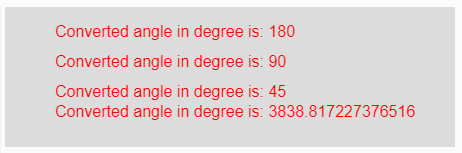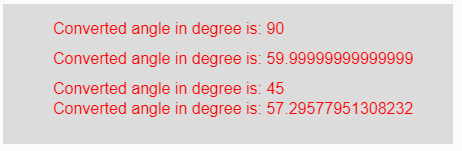# p5.js | degrees() function

The degrees() function in p5.js is used to convert a given radian measurement value to its corresponding value in degrees.

Syntax:

`degrees( radian )`

Parameters: This function accepts single parameter radian which is to be converted into degrees.

Return Value: It returns the converted angle into degrees.

Below programs illustrate the degrees() function in p5.js:

Example 1: This example uses degrees() function to convert given radian measurement value to its corresponding value in degrees.

 `function` `setup() {  ` `  `  `    ``// Creating Canvas size ` `    ``createCanvas(450, 140);  ` `}  ` ` `  `function` `draw() {  ` `      `  `    ``// Set the background color  ` `    ``background(220);  ` `    `  `    ``// Initializing some angles in radians ` `    ``let Rad1 = PI;  ` `    ``let Rad2 = PI / 2;  ` `    ``let Rad3 = PI / 4;  ` `    ``let Rad4 = 67;  ` `     `  `     `  `    ``// Calling to degrees() function ` `    ``let A = degrees(Rad1); ` `    ``let B = degrees(Rad2); ` `    ``let C = degrees(Rad3); ` `    ``let D = degrees(Rad4); ` `     `  `    ``// Set the size of text  ` `    ``textSize(16);  ` `      `  `    ``// Set the text color  ` `    ``fill(color(``'red'``));  ` `    `  `    ``// Getting converted angles into degree ` `    ``text(``"Converted angle in degree is: "` `+ A, 50, 30); ` `    ``text(``"Converted angle in degree is: "` `+ B, 50, 60); ` `    ``text(``"Converted angle in degree is: "` `+ C, 50, 90); ` `    ``text(``"Converted angle in degree is: "` `+ D, 50, 110); ` `}  `

Output:Example 2: This example uses degrees() function to convert given radian measurement value to its corresponding value in degrees.

 `function` `setup() {  ` `  `  `    ``// Creating Canvas size ` `    ``createCanvas(450, 140);  ` `}  ` ` `  `function` `draw() {  ` `      `  `    ``// Set the background color  ` `    ``background(220);  ` `     `  `    ``// Calling to degrees() function with different ` `    ``// radians value as parameter ` `    ``let A = degrees(PI/2); ` `    ``let B = degrees(PI/3); ` `    ``let C = degrees(PI/4); ` `    ``let D = degrees(PI/PI); ` `     `  `    ``// Set the size of text  ` `    ``textSize(16);  ` `      `  `    ``// Set the text color  ` `    ``fill(color(``'red'``));  ` `    `  `    ``// Getting converted angles into degree ` `    ``text(``"Converted angle in degree is: "` `+ A, 50, 30); ` `    ``text(``"Converted angle in degree is: "` `+ B, 50, 60); ` `    ``text(``"Converted angle in degree is: "` `+ C, 50, 90); ` `    ``text(``"Converted angle in degree is: "` `+ D, 50, 110); ` `}   `

Output:Reference: https://p5js.org/reference/#/p5/degreesMy Personal Notes arrow_drop_upCheck out this Author's contributed articles.

If you like GeeksforGeeks and would like to contribute, you can also write an article using contribute.geeksforgeeks.org or mail your article to contribute@geeksforgeeks.org. See your article appearing on the GeeksforGeeks main page and help other Geeks.

Please Improve this article if you find anything incorrect by clicking on the "Improve Article" button below.

Article Tags :

Be the First to upvote.

Please write to us at contribute@geeksforgeeks.org to report any issue with the above content.